# Maths - Groupoid

There are different ways to define a groupoid such as:

• Algebraic definition: same definition as a group but with a partial function replacing the binary operation.
• Categorical definition: a category in which every morphism is invertible.
• As an oriented graph (if <x,y> is an edge then <y,x> is not).

### Applications

Groupoids form the underlying structure in homotopy type-theory (HoTT) Discussed on the page here.

### n-Groupoids

Level In Type Theory

Represents Equality in Hott

see page here

-2-Groupoid

contractible singleton

(Unit Type)

-1-Groupoid

subsingleton

(Mere proposition)0-Groupoid (n-lab) Set Example - equality in Natural Numbers1-Groupoid (n-lab)   Example - equality in Monoid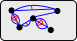2-Groupoid (n-lab)   Example - equality in Category

## Groupoids and Equivalences

Group Definition Equivalence Definition
Identity a = a•Id = Id •a

#### reflexive

a~a

Reverse a•inv(a) = inv(a)•a = Id

#### symmetric

if a~b then b~a

Associatively a•(b•c) = (a•b)•c

#### transitive

if a~b and b~c then a~c

A groupoid is a setoid with natural coherence axioms. A setoid is a set with an equivalence relation.

Another special case is G-sets, sets with an action of a group G.

### Groupoids and Type Theory

Type Theory Groupoids

a,b : A
p,q : a=b
α,β : p=q
...More about type theory on page here.

### Example 1 - Fifteen Puzzle

 There used to be a puzzle, sometimes called the 15 puzzle, consisting of 15 sliding plastic tiles in a 4 by 4 frame. There is only one space without a tile, so the only moves possible are to slide the adjacent tiles into that space. The tiles have letters or numbers on them and the goal is to get them in a certain order.So for the first move, in this starting position, there are only 2 moves possible: move tile 1 into the space move tile 4 into the space Say we move tile 1:Now there are 3 moves possible: move tile 1 into the space (inverse of first move) move tile 2 into the space move tile 5 into the spaceThis example illustrates the different ways of defining the groupoid:

 We could think of the position of all the tiles as being its state. There is then a mapping from state to state, but this mapping is partial because only certain moves will be possible depending on where the space is.Rather than one set of endo functions we could have a set of states, one for each possible location of the space. So now instead of the functions being endofunctions they go between these states. These functions are no longer partial but they are still permutations.So we could specify the state of the game by:

• The starting position.
• Together with the sequence of positions of the holes.

Since all arrows are reversible we can always get back to a previous state by retracing a path back. However, if we start with a hole in a particular place and then follow a circular path (for the hole) so the hole ends up in the same place the state (position of tiles) may change so this is not equal to Id.

## Categorical Definition

Categorical Definition Groupoid Definition
Identity Arrow from all objects to themselves that do not change it. Identity element exists
Reverse Arrows not generally reversible.

All arrows can be reversed.

f • f-1 = Id

Associatively Composition of morphisms Associatively

A groupoid is a category in which every morphism is invertible. So this means that, when objects are connected by arrows, they are Isomorphic. However, just because the objects are isomorphic, does not mean the structure is not complicated as we saw with the 15-puzzle.

If the category is based on an underlying set then we can think of the arrows as permutations.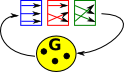In an ordinary group (represented a permutation group) the functors are endofunctors with only a single object.In a groupoid the permutations can go between objects. Because the mappings are reversible the objects are isomorphic, we can get back to Id, however we don't have to use the reverse so its possible to use the round trip to permute the elements.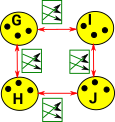Its possible to have permutations such that we can only permute the elements if we go round the loop but not if we reverse the path. Depending on the complexity of the groupoid there may be multiple paths round the loop which produce the same permutation so we have possible equivalences between paths. That is, higher order equivalences.

## ω-groupoid

An ω-groupoid is an ω-category in which all higher morphisms are equivalences.

A ω-category (or ∞-category) is a higher order category.

• all composition operations are strictly associative.
• all composition operations strictly commute with all others (strict exchange laws).
• all identity higher morphisms are strict identities under all compositions.

## Fundamental Groupoid - Topology

The fundamental groupoid of a space is a groupoid with:

• Objects - points of a space.
• Morphisms - paths in X, identified up to endpoint-preserving homotopy.
ω-groupoid topological space
type space
term point
path(x,y)

Id(x,y)

paths from x to y in the space.

Also see fundamental groupoid on page here.

### Example 2 - Computational Path

Here we are looking at the relationship between two types of equality: definitionally equality and propositional equality. These are explained on this page.

 In Nat consider 'n', '0+n' and 'n+0' they are equal terms in some way. 'n' and '0+n' are definitionally equal because they automatically normalise to the same. Definitionally equality is denoted with a 3-bar equals sign (≡) or just by using the same symbol. But 'N+0' is propositionally (but not definitionally) equal to N. It is denoted with a normal equals sign (=) and shown as an arrow between nodes.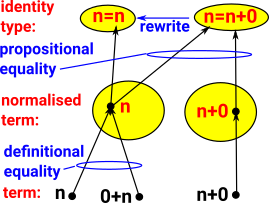This can get more complicated when we have types involving containers within containers.## Groupoid in Type Theory

• Used in Homotopy Type Theory (HoTT) see page here.

## Computer Model of Groupoid

A model of a groupoid might contain a hierarchy of types:

• base : Type
• path : base -> base
• path2 : path -> path
• path3 : path2 -> path2

So the easiest base type to think about might be, for instance, a finite set. In that case a path would be the possible equalities between the elements of the set. This is a reversible mapping which is a permutation.

What would path2 look like? That is a mapping between permutations.

In the 15-puzzle example there is only one mapping (path) at most, between any two nodes. So the higher path is just a trivial Id mapping, then its trivial mappings all the way up. So that doesn't give us any intuition about higher level paths. Perhaps if we added some ability to switch routes (like a railway switching game?) would that do it?

## Oidification

Oidification allows us to start with a single object and one (or more) arrows back to itself and generalise this to multiple objects. This new structure does not need to have arrows between every pair of objects but composition always exists and every object has an identity arrow.

Here the words 'object' and 'arrow' are used in the category theory sense as described on the page here.

Here are some examples:

Single Object Multiple Objects

Setoid

This imposes an external equivalence relation and internalises it.The single object needs to have the reflexive relation, that is, be equal to itself.

An example would be a set with a set with a fixed number of elements.Since the arrows are reflexive, symmetric and transitive this gives an equivalence relation.

Groupoid

This can be thought of as a weakening of a setiod. Instead of equivalences we have isomorphisms, that is the permutations show how objects are equal in multiple ways.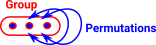Category

Just to confuse things the naming conventions have changed here. Monoid has an 'oid' but this is the single object case. A Monoidoid is a category.## Implement in Idris

Is it possible to implement a groupoid using Idris?

I was thinking about something like this:

 ```||| Groupoid where base type is a ||| finite set (implemented as a list) record Groupoid (level : Nat) where constructor MkGroupoid source : Nat dest : Nat Path : List (Groupoid (level + 1)) fifteenPuzzle : Groupoid 0 fifteenPuzzle = let c1:Groupoid 2 = MkGroupoid 1 2 [] c2:Groupoid 2 = MkGroupoid 2 1 [] swap = [c1,c2] b1_2:Groupoid 1 =MkGroupoid 1 2 swap b1_5:Groupoid 1 =MkGroupoid 1 5 swap b2_3:Groupoid 1 =MkGroupoid 2 3 swap b2_6:Groupoid 1 =MkGroupoid 2 6 swap b3_4:Groupoid 1 =MkGroupoid 3 4 swap b3_7:Groupoid 1 =MkGroupoid 3 7 swap b4_8:Groupoid 1 =MkGroupoid 4 8 swap b5_6:Groupoid 1 =MkGroupoid 5 6 swap b5_9:Groupoid 1 =MkGroupoid 5 9 swap b6_7:Groupoid 1 =MkGroupoid 6 7 swap b6_10:Groupoid 1 =MkGroupoid 6 10 swap b7_8:Groupoid 1 =MkGroupoid 7 8 swap b7_11:Groupoid 1 =MkGroupoid 7 11 swap b8_12:Groupoid 1 =MkGroupoid 8 12 swap b9_10:Groupoid 1 =MkGroupoid 9 10 swap b9_13:Groupoid 1 =MkGroupoid 9 13 swap b10_11:Groupoid 1 =MkGroupoid 10 11 swap b10_14:Groupoid 1 =MkGroupoid 10 14 swap b11_12:Groupoid 1 =MkGroupoid 11 12 swap b11_15:Groupoid 1 =MkGroupoid 11 15 swap b12_16:Groupoid 1 =MkGroupoid 12 16 swap b13_14:Groupoid 1 =MkGroupoid 13 14 swap b14_15:Groupoid 1 =MkGroupoid 14 15 swap b15_16:Groupoid 1 =MkGroupoid 15 16 swap in the (Groupoid 0) (MkGroupoid 0 0 [b1_2,b1_5,b2_3,b2_6,b3_4,b3_7,b4_8, b5_6,b5_9,b6_7,b6_10,b7_8,b7_11,b8_12, b9_10,b9_13,b10_11,b10_14,b11_12,b11_15,b12_16, b13_14,b14_15,b15_16])```